# 23Making maps in R

Biologists need almost as many maps as geographers, perhaps to show where our field sites are, the range of a species, or the migration path of a bird.

Typically, the data we want to show is added to a base map. These can be added to different types of base map

• vector map showing the coastline, and perhaps political borders, rivers and other features

Terminology

Small scale vs large scale? The terminology is confusing!

Map scales are given as a ratio. A world map might have a scale of 1:100000000. 1 cm on the map represents 100000000 cm on the ground - a ratio of 1/100000000. A small number hence a small-scale map.

Conversely, a map of a city might have a scale of 1:25000. 1 cm on the map represents 25000 cm on the ground - a ratio of 1/25000. A large number hence a large-scale map.

Large-scale maps are usually high resolution and cover a small spatial extent.

## 23.1 Vector base maps

### 23.1.1`rnaturalearth`

The `rnaturalearth` package makes Natural Earth data available. Natural Earth features include coastlines, rivers, bathymetry, political borders, roads and railways at scales 1:10m, 1:50m, and 1:110 million. The low-resolution (1:110 million) coastline and country data, suitable for world maps, are included in the `rnaturalearth` package. The `rnaturalearthdata` package, also on CRAN, contains medium-resolution data. High-resolution (1:10 million) data are in the `rnaturalearthhires` package, instructions for installing this are below.

``````library(rnaturalearth)

world <- ne_countries(scale = 110, returnclass = "sf")
small_scale_map <- ggplot() +
geom_sf(data = world) +
coord_sf(xlim = c(-20, 50), ylim = c(33, 80)) +
ggtitle("Europe")

europe <- ne_countries(scale = 50, returnclass = "sf", continent = "Europe")
medium_scale_map <- ggplot() +
geom_sf(data = europe) +
coord_sf(xlim = c(5, 30), ylim = c(55, 71)) +
ggtitle("Norden")

# Need extra package for high resolution data
# install.packages("rnaturalearthhires", repos = "https://ropensci.r-universe.dev")

norway <- ne_countries(scale = 10, returnclass = "sf", country = "Norway")

large_scale_map <- ggplot() +
geom_sf(data = norway) +
coord_sf(xlim = c(4, 9), ylim = c(59, 62)) +
ggtitle("Vestland")

# combine maps with patchwork
library(patchwork)
small_scale_map + medium_scale_map + large_scale_map``````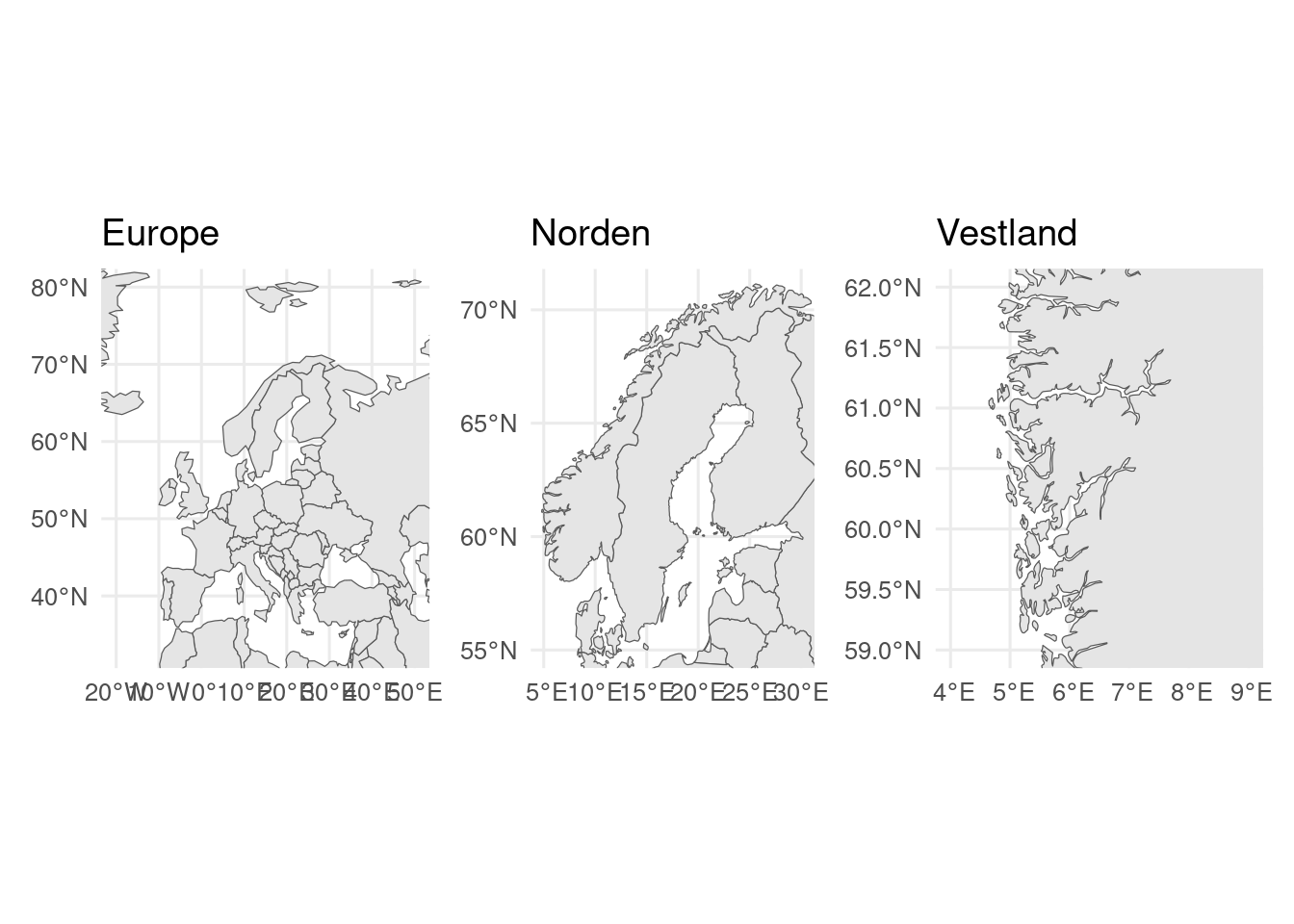`coord_sf()` is used to show only part of the map.

`sf` and `sp` packages

`sf` and `sp` are both packages for geospatial data. `sf` is the newer package that supports the “simple features” standard and is what I strongly recommend.

Rivers and lakes

Sometimes the coastline and national borders are sufficient. Sometimes they aren’t very informative and you want to add more features, such as rivers, lakes and cities. This can be done with data from Natural Earth (or other sources). Natural Earth datasets can be downloaded directly from the website or with `ne_download()`

``````# download if needed
if(!file.exists("maps/ne_10m_rivers_europe.shp")){
destdir = "maps/", load = FALSE) # major rivers
destdir = "maps/", load = FALSE) # major lakes
}

rivers <- ne_load(scale = 10, type = "rivers_lake_centerlines", destdir = "maps", returnclass = "sf")
lakes <- ne_load(scale = 10, type = "lakes", destdir = "maps", returnclass = "sf")

ggplot() +
geom_sf(data = europe) +
geom_sf(data = rivers, colour = "blue", linewidth = 0.2) +
geom_sf(data = lakes, fill = "lightblue") +
coord_sf(xlim = c(5, 30), ylim = c(55, 71))``````

Extra rivers and lakes for Europe and N. America are available from Natural Earth, but for a large-scale map, other data sets may be better, for example from Noregs vassdrags- og energidirektorat.

### 23.1.2`ggOceanMaps`

`ggOceanMaps` is, as the name suggests, focused on ocean map, with coastlines, bathymetry and also glaciers. `ggOceanMaps` requires `ggOceanMapsData`, which needs to be installed separately. You do not need to load `ggOceanMapsData` wih `library()`

``remotes::install_github("MikkoVihtakari/ggOceanMapsData")``

Now `ggOceanMaps` is ready to use.

``````library(ggOceanMaps)
#limits are given longitude min/max, latitude min/max
basemap(limits = c(-30, 30, 50, 80),
bathymetry = TRUE,
glaciers = TRUE)``````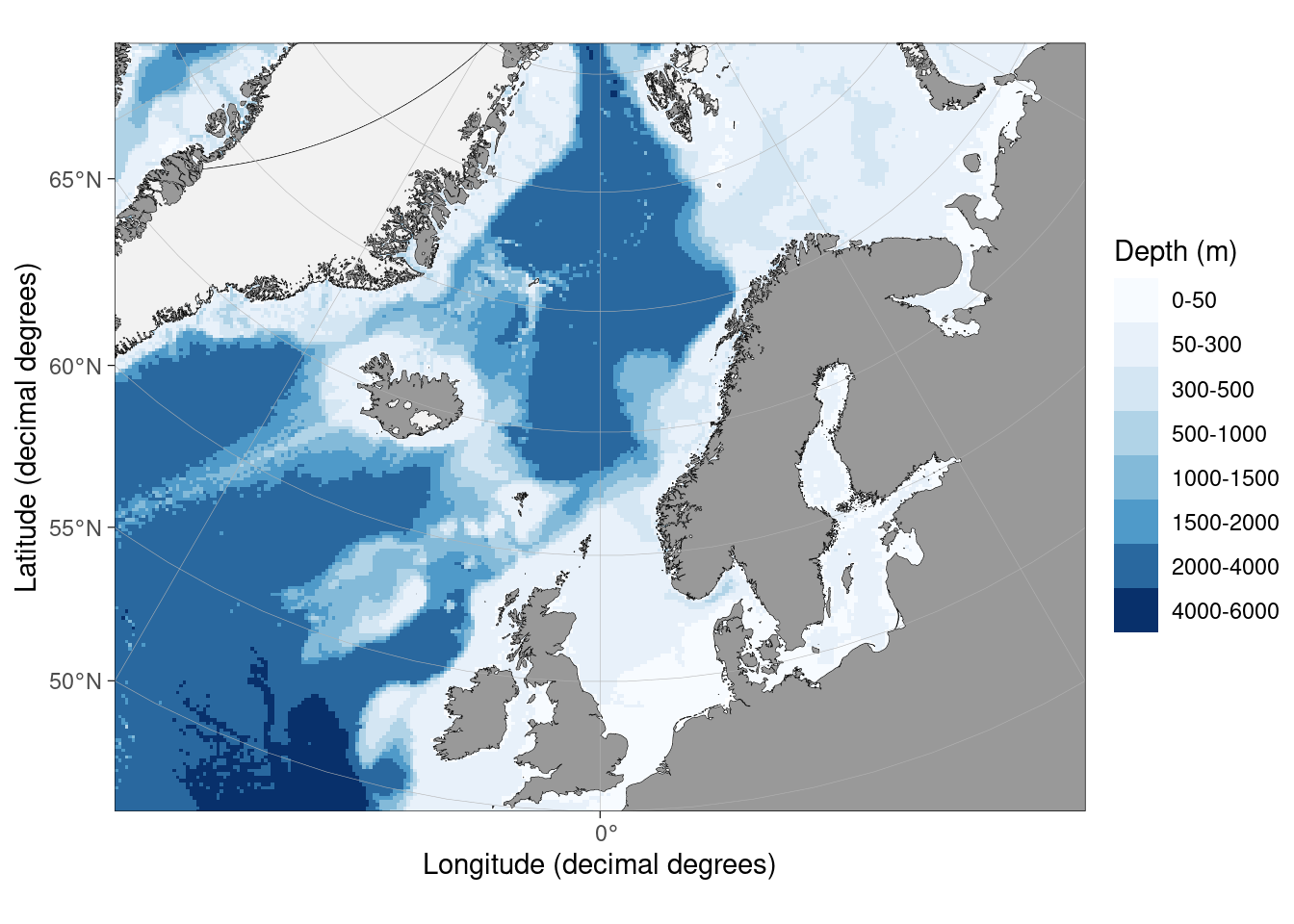Exercise

Make a map of Svalbard using either `rnaturalearth` or `ggOceanMaps`.

### 23.1.3 Other vector files

The maps in `rnaturalearth` and `ggOceanMaps` are good and the global and regional scale, but lack resolution for local scale maps, and may lack features we are interested in.

For such maps we need to find alternative resources. These could be a shapefile, GeoJSON or GeoPackage file, all of which can be imported with `sf::st_read()`.

Shapefiles

A “shapefile” is not one file but collection of several files in the same directory, only of which has the extension “.shp”.

Good sources of data for Norway include:

This is a map of the fylke of Norway.

``library(sf)``
``Linking to GEOS 3.6.2, GDAL 2.2.3, PROJ 4.9.3; sf_use_s2() is TRUE``
``````# https://kartkatalog.geonorge.no/metadata/norske-fylker-og-kommuner-illustrasjonsdata-2021-klippet-etter-kyst/f08fca3c-33ee-49b9-be9f-028ebba5e460
# This map will be out of date when fylker are reorganised
``````Reading layer `fylker2021' from data source
`/home/gbsrt/Documents/teaching/biostats-1/WorkingInR/data/fylker2021.json'
using driver `GeoJSON'
Simple feature collection with 11 features and 2 fields
Geometry type: MULTIPOLYGON
Dimension:     XY
Bounding box:  xmin: -77298.97 ymin: 6448400 xmax: 1115097 ymax: 7939977
Projected CRS: 25833``````
``````ggplot(fylker) +
geom_sf()``````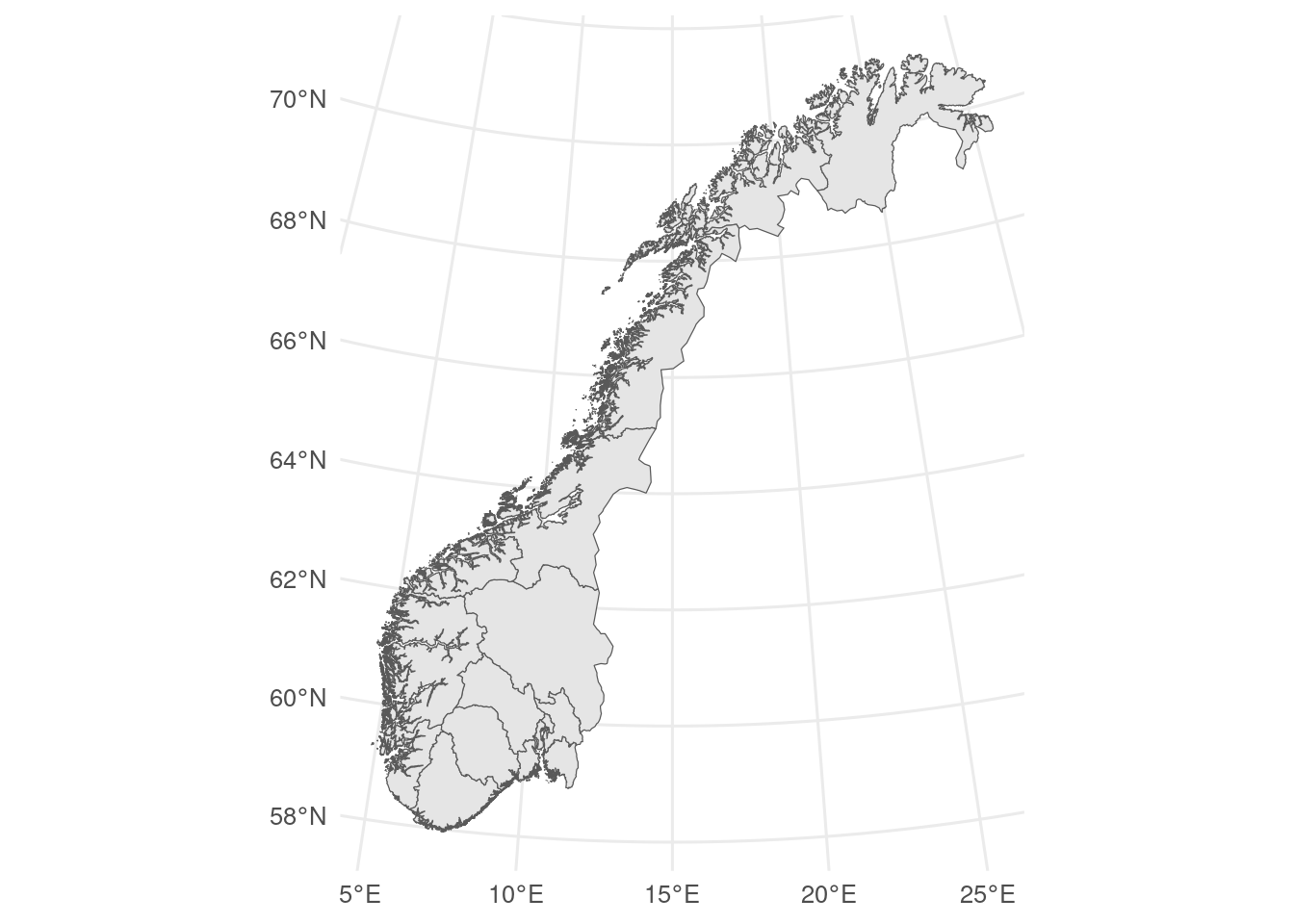Coordinate reference systems

Most geographic data are given with latitude and longitude, but sometimes, especially for local-regional maps, the data are given as Universal Transverse Mercator (UTM) coordinates instead.

UTM coordinates are a projection of the spherical Earth onto one of 60 flat surfaces.

Most modern latitude-longitude data will use the WGS84 geodetic standard. Older data might use other standards.

You can find the coordinate system of a `sf` class object with `sf::st_crs()`.

``sf::st_crs(fylker)``
``````Coordinate Reference System:
User input: 25833
wkt:
PROJCS["ETRS89 / UTM zone 33N",
GEOGCS["ETRS89",
DATUM["European_Terrestrial_Reference_System_1989",
SPHEROID["GRS 1980",6378137,298.257222101,
AUTHORITY["EPSG","7019"]],
TOWGS84[0,0,0,0,0,0,0],
AUTHORITY["EPSG","6258"]],
PRIMEM["Greenwich",0,
AUTHORITY["EPSG","8901"]],
UNIT["degree",0.0174532925199433,
AUTHORITY["EPSG","9122"]],
AUTHORITY["EPSG","4258"]],
PROJECTION["Transverse_Mercator"],
PARAMETER["latitude_of_origin",0],
PARAMETER["central_meridian",15],
PARAMETER["scale_factor",0.9996],
PARAMETER["false_easting",500000],
PARAMETER["false_northing",0],
UNIT["metre",1,
AUTHORITY["EPSG","9001"]],
AXIS["Easting",EAST],
AXIS["Northing",NORTH],
AUTHORITY["EPSG","25833"]]``````

This gives a lot of information, the most important is that the coordinate reference systems is UTM zone 33N.

If we need to change a coordinate reference systems, we can do that with `sf::st_transform()`. You need to know the EPSG code of the target reference system, or the wkt. The EPSG code for WGS84 is 4326.

``fylker2 <- sf::st_transform(fylker, crs = 4326)``

`geom_sf()` will automatically transform coordinate systems (if they are specified).

## 23.2 Tiled basemaps

Tiled basemaps can be used with either `ggspatial` or `ggmap` packages. I recommend using `ggspatial` as it is consistent with the other mapping tools used here.

If you use a tiled map background, you should attribute the source (e.g., “Copyright OpenStreetMap contributors” when using an OpenStreetMap-based tiles).

### 23.2.1`ggspatial`

We can add a tiled-basemap to a plot with `annotation_map_tile()` (you may need to install some extra packages at this stage - R will tell you). Here, we need to use `coord_sf()` to set the map extent and coordinate reference system as we have not added any `sf` layers with `geom_sf()`. Downloaded tiles will be stored in the maps directory (which you may need to make first).

``````library(ggspatial)
ggplot() +
annotation_map_tile(
type = "osm",
cachedir = "maps/",
zoomin = -1) + # sets the zoom level relative to the default
coord_sf(
xlim = c(4.5, 6),
ylim = c(60.5, 61),
crs = 4326) # EPSG code for WGS84``````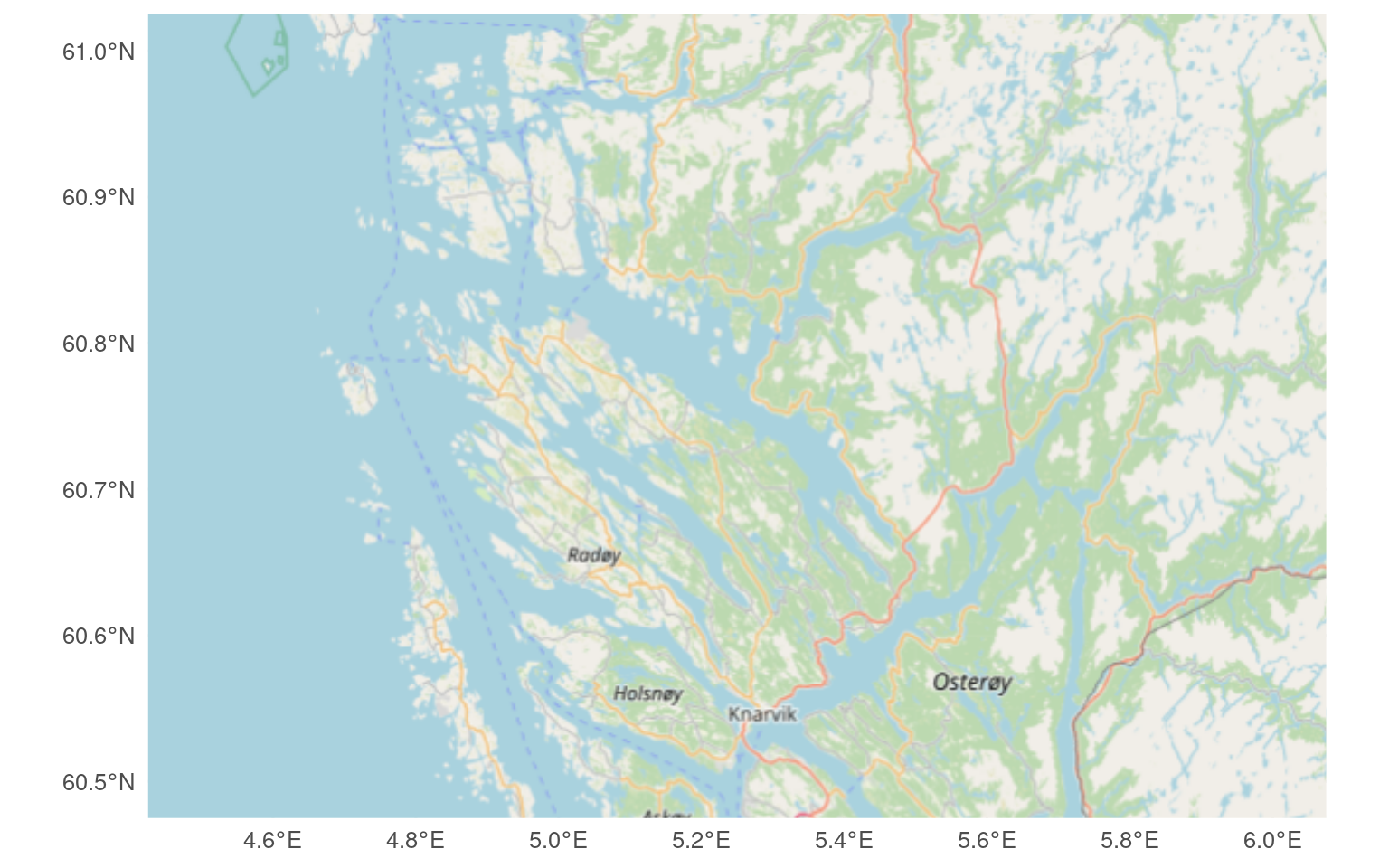Several different types of maps are available (see `rosm::osm.types()`) and more can be added.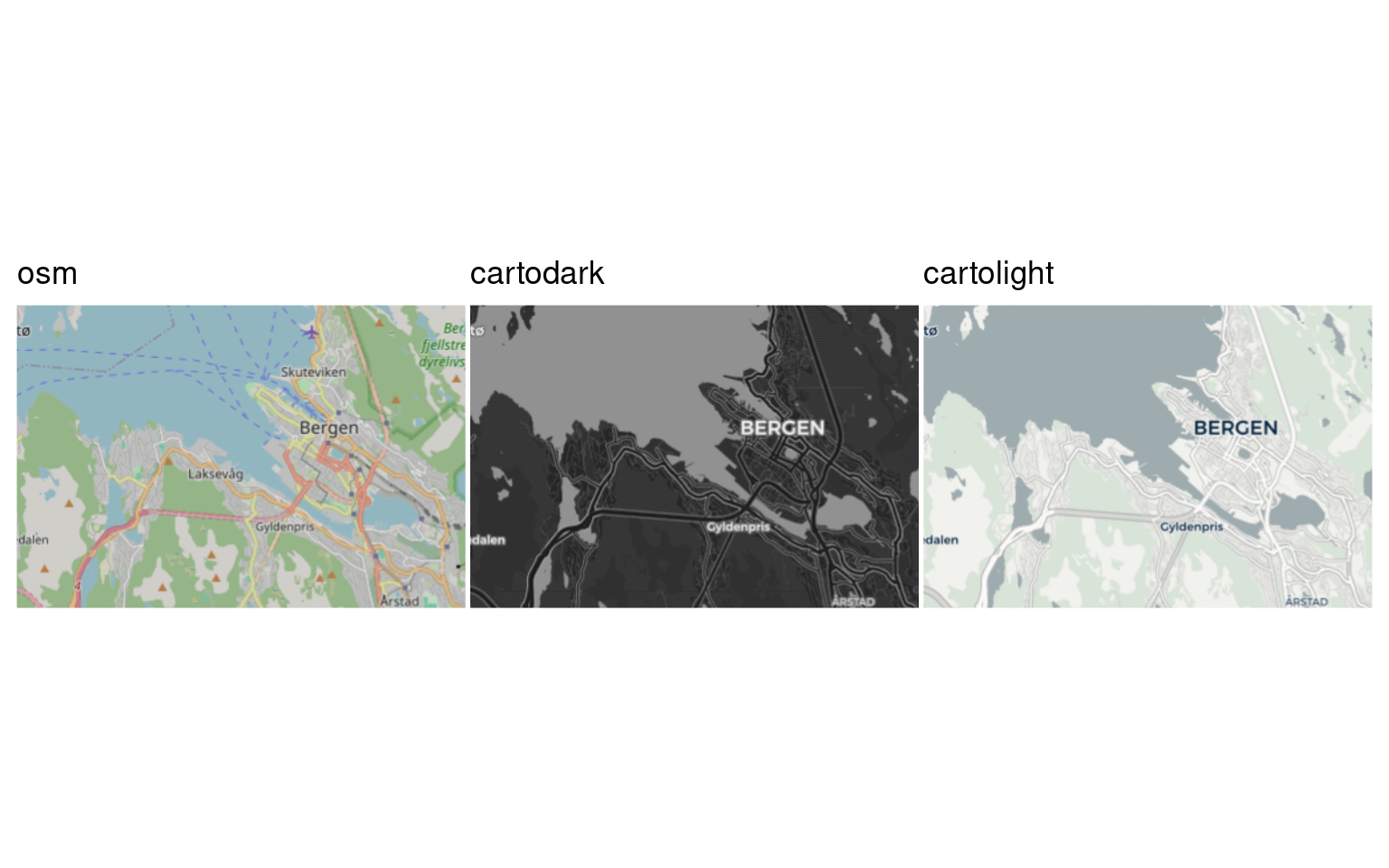### 23.2.2`ggmap`

The `ggmap` package lets you use Google Maps and other similar maps as a basemap.

`ggmap` can use maps and satellite image from Google, but you need to register for an API key. You shouldn’t be charged unless you make a lot of maps (thousands per month).

``````library(ggmap)

bergen <- get_map(
location = c(5.24, 60.37, 5.36, 60.41), #  left/bottom/right/top
source = "stamen"
)
ggmap(bergen)``````Maps made with a tiled background can appear cluttered with unnecessary information. They are probably best for small areas.

Exercise

Make a tiled map that shows your favourite holiday destination.

## 23.3 Adding data to the basemap

After deciding what type of base map to draw, we can add the data we want to show with the map. This can be

• points, line, and polygons

• Shaded political units (a cloropleth map)

• A grid of values (raster)

### 23.3.1 points/lines/polygons

Points lines and polygons can be added to the base map. If the data are already a `sf` object they can be plotted with `geom_sf()`.

``````# aquaculture sites downloaded from Barentswatch.no/fiskinfo
``````Reading layer `flate-ihht-akvakulturregisteret20220928' from data source
`/home/gbsrt/Documents/teaching/biostats-1/WorkingInR/data/flate-ihht-akvakulturregisteret20220928.geojson'
using driver `GeoJSON'
Simple feature collection with 1330 features and 4 fields
Geometry type: MULTIPOLYGON
Dimension:     XY
Bounding box:  xmin: 4.660283 ymin: 58.01472 xmax: 30.41932 ymax: 71.02117
Geodetic CRS:  4326``````
``````# with rnaturalearth
ggplot() +
geom_sf(data = europe) +
geom_sf(data = aquaculture, colour = "red") +
coord_sf(xlim = c(5, 30), ylim = c(55, 71))  ``````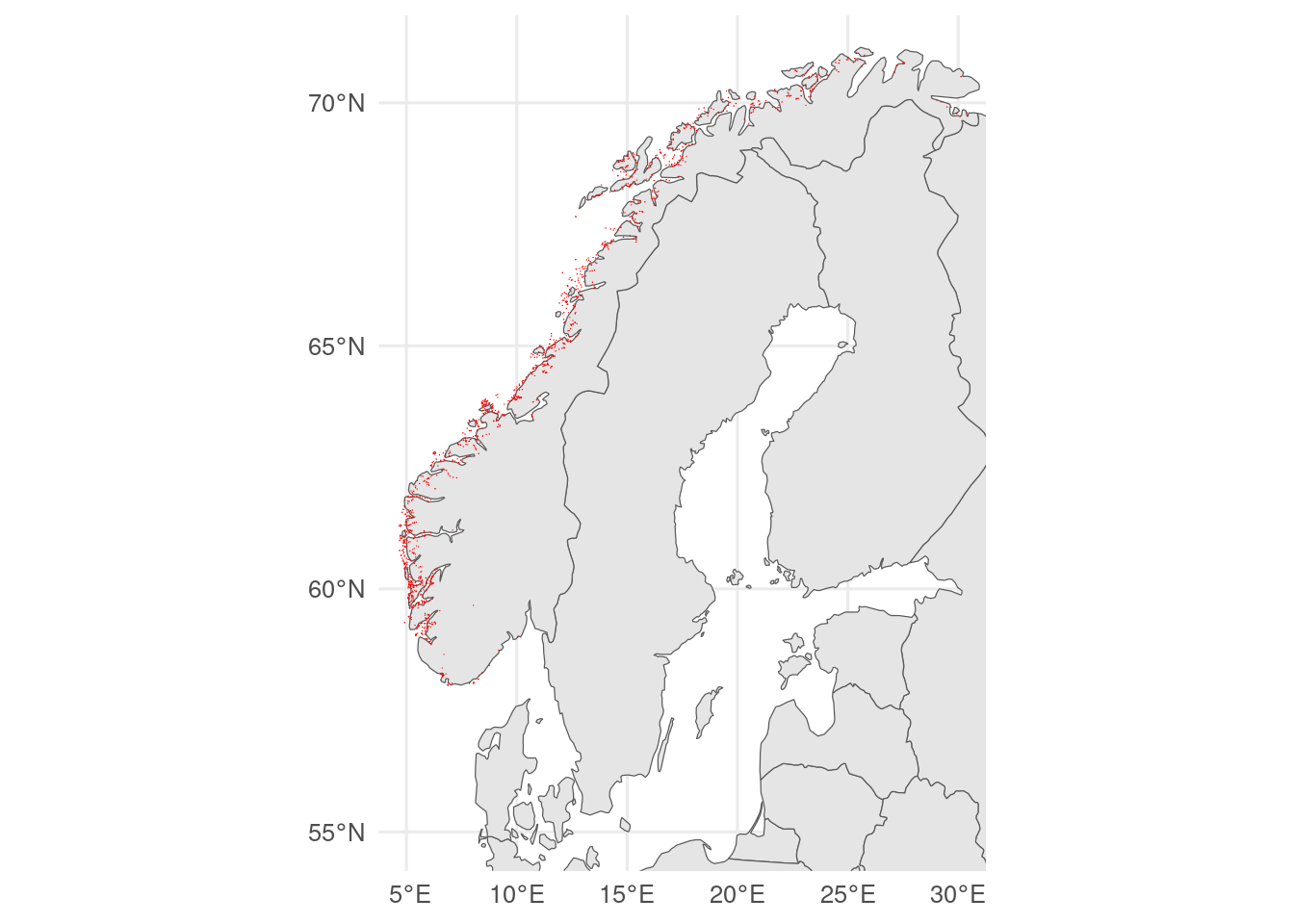``````# with ggOceanMaps

basemap(limits = c(-30, 30, 50, 80)) +
geom_sf(data = aquaculture, colour = "red")``````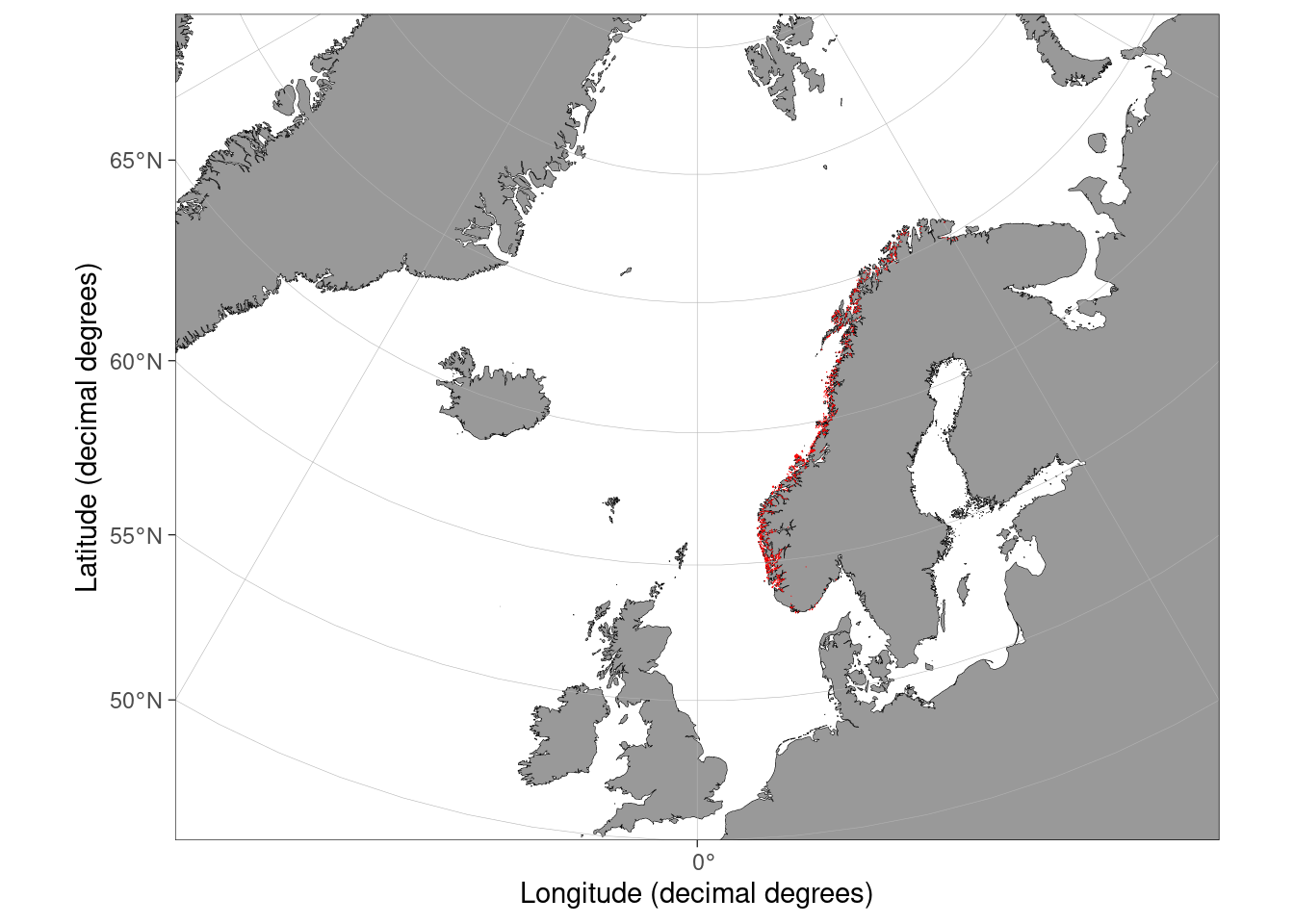``````# with ggmap
vestland <- get_map(
location = c(4,  59, 8, 62),
source = "stamen"
)

# needs inherit.aes = FALSE
ggmap(vestland) +
geom_sf(data = aquaculture, colour = "red", inherit.aes = FALSE, linewidth = 1) # thicker lines to make visible``````Alternatively, you can make/import a tibble with the geographic data and add them to the basemap with the relevant spatially aware geom. So `geom_spatial_point()` rather than `geom_point()` and `geom_spatial_path()` rather than `geom_path()`.

``````library(ggspatial)

locale = locale(decimal_mark = ",")) |>
janitor::clean_names()

# ggOceanMaps

basemap(data = osprey) +
geom_spatial_path(aes(x = longitude_e, y = latitude_n, colour = speed),
data = osprey, linewidth = 1) +
labs(colour = expression(Speed~km~h^{-1}))``````

`geom_spatial_point()` assumes that the data are latitude-longitude coordinates. It they are UTM, you will need to use the `crs` argument with the correct EPSG code.

geom_path() vs geom_line()

`geom_path()` draws a line from the first point in the dataset to the second and so on. This is useful for plotting on maps with `geom_spatial_path()`.

`geom_line()` draws a line from the left-most point to the next left-most point in the dataset. This is useful for plotting timeseries.

Degrees minutes and seconds

For latitude-longitude data, we recommend using decimal degrees (Bergen is at 60.3807°N, 5.3323°E). But archived data can be in all sorts of unhelpful formats, such as degrees minutes and seconds (Bergen is at 60° 22’ 50.52” N 5° 19’ 56.28” E). If you get data like this, you need to convert it to decimal degrees. The `parzer` package can help (it is like `lubridate` for latitude-longitude data). For example:

``parzer::parse_lat("60° 22' 50.52''N")``
`` 60.3807``
Exercise

Otters (Lutra lutra) have re-established in Vestland. File ExcelExport_7972978_Page_1a.xlsx, (from https://artsobservasjoner.no, edited to remove an invalid unicode character) shows observations of otters in Vestland. Make a map of the observations. Relevant columns are “East Coord”, “North Coord”. The coordinates are UTM zone 33N, EPSG code 25833.

### 23.3.2 Cloropleth maps

Cloropleth maps are useful for plotting data that have been aggregated to a geographic unit (kommune, fylke, country etc). A `sf` object is a special type of data frame that we can `filter()`, `mutate()` or `left_join()` to other data frames. We need a tibble with the data that we can join to the `sf` object with the geographic units. Here, I use data on 2021 phosphorous discharge in municipal wastewater from SSB and join it by Fylkesnummer, and plot it by setting `fill` in the `aes`.

``````p_discharge <- readxl::read_excel(path = "data/05280_20230421-012359.xlsx",
skip = 4, n_max = 11) |>
janitor::clean_names() |>
separate_wider_regex(
cols = x1, # split fist column
patterns = c(Fylkesnummer = "\\d{2}", " ", Fylkesnavn = ".*"))# using regular expressions
# \\d{2} = 2 numbers, " " = space, ".*" = any number of any character, ie everything else

# join to fylker
fylker_discharge <- fylker |>
left_join(p_discharge, by = "Fylkesnummer")

ggplot(fylker_discharge) +
geom_sf(aes(fill = total_discharge)) +
labs(fill = "Wastewater\nP discharge\n(tonnes)")``````
Cartograms

Sometimes cloropleth maps can be misleading. An alternative is to warp space so that the area of each region is proportional to the value. This is a cartogram, which can be made with the `cartogram` package.

``````library(cartogram)

# simplify sf object for speed
fylker_discharge_sim <- sf::st_simplify(fylker_discharge, dTolerance = 1000)

# make cartogram
kart <- cartogram_cont(fylker_discharge_sim, weight = "total_discharge", itermax = 5)

# plot cartogram
ggplot(kart) +
geom_sf(aes(fill = total_discharge)) +
labs(fill = "Wastewater\nP discharge\ntonnes") +
theme(legend.position = c(.99, .01),
legend.justification = c(1, 0))``````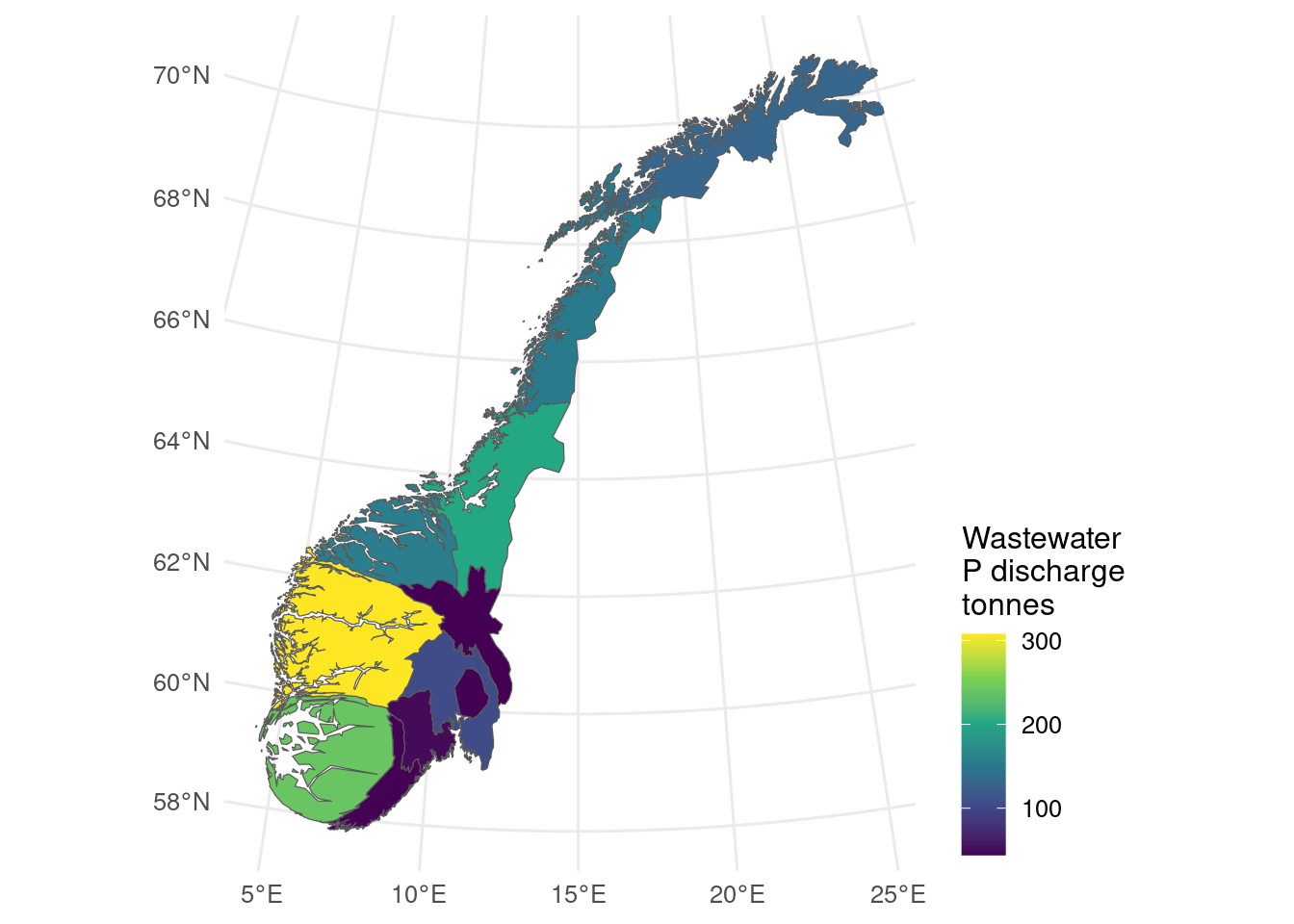Figure 23.4: Cartogram showing total phosphate discharge in municipal wastewater by fylke.
Exercise

With `rnaturalearth` data, make a world map that shows the population (column pop_est) of each country.

### 23.3.3 Rasters

Rasters can be used to show maps of continuous data, for example, elevation or sea surface temperature, or model predictions. Depending on the extent, you might not need a separate basemap.

### 23.3.4`terra`

The `terra` package can import raster images in several formats, including GeoTIFF.

`terra` vs `raster` vs `stars` packages

The `terra` package is an update to the widely-used `raster` package. It should be faster and easier to use. `terra` can integrate with `ggplot2` with `tidyterra`.

`stars` is designed for spatio-temporal arrays. There are some things it cannot do that `terra` can (and vice versa). It has good integration with `sf` and `ggplot2`.

Rasters imported with `terra` are easy to plot with the base R `plot()` function, but if we want to use `ggplot()`, it is easiest to use a geom from the `tidyterra` package.

``````library(terra)
library(tidyterra)

# import digital elevation model
# data from https://topotools.cr.usgs.gov/gmted_viewer/viewer.htm
norway_dem <- rast("data/50N000E_20101117_gmted_med300.tif")

coast_vector <- fylker |>
# transfrom to crs of raster
st_transform(crs = terra::crs(norway_dem)) |>
# convert to spatVector
vect()

# crop to vestland and rename the data layer
vestland_extent <- ext(4.5, 9, 59, 62)
vestland_dem <- crop(norway_dem, vestland_extent) |>
rename(Elevation = `50N000E_20101117_gmted_med300`)

# plot
ggplot() +
geom_spatraster(data = vestland_dem) +
scale_fill_viridis_c(na.value = "grey90") +
scale_x_continuous(expand = c(0, 0)) +
scale_y_continuous(expand = c(0, 0)) +
labs(fill = "Elevation, m")``````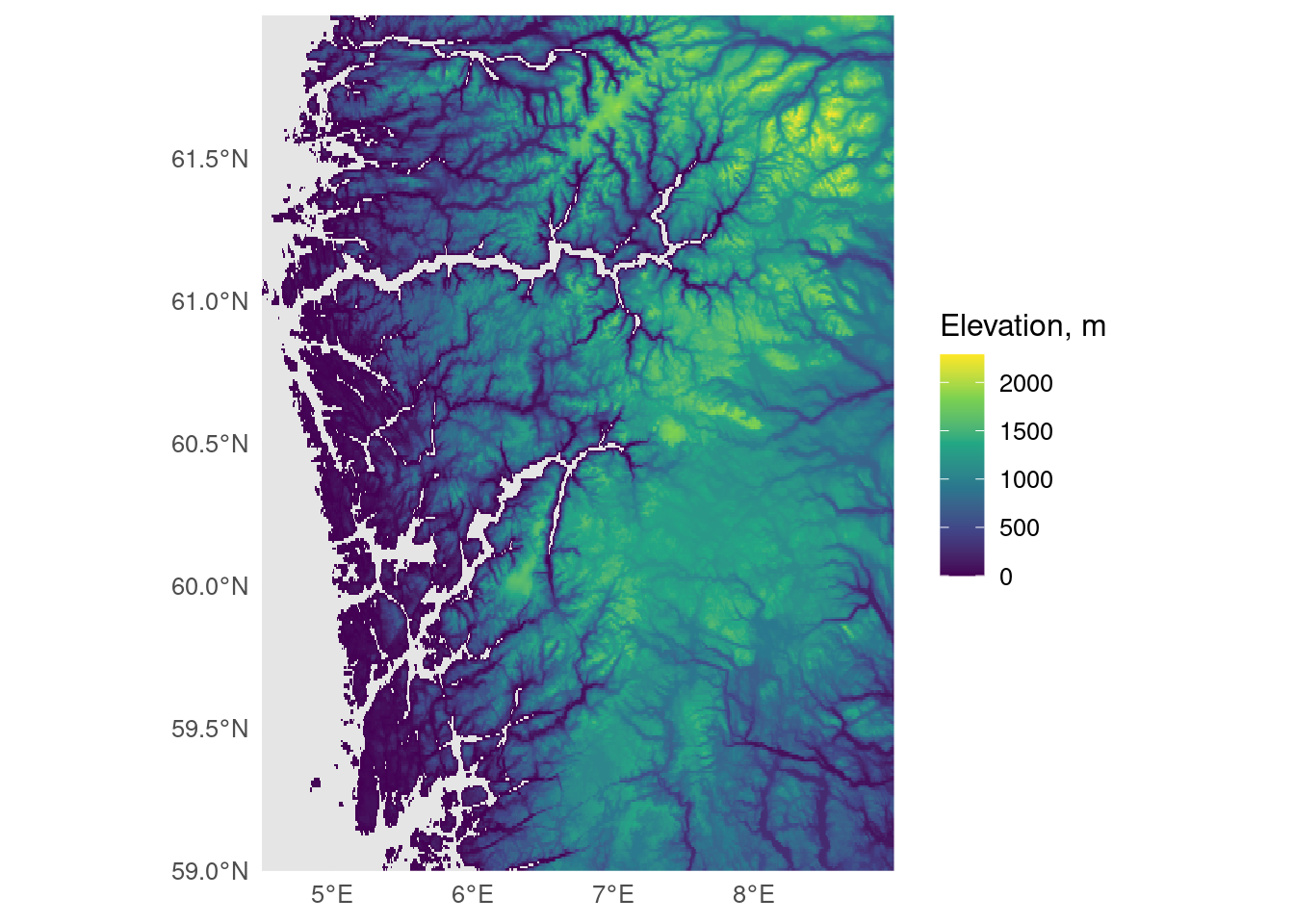## 23.4 Scalebars, north pointer etc

Scalebars and north pointers can be added with the `ggspatial` package or the `ggsn` package. North points are not always very useful if the map has gridlines as these already indicate north. A scalebar can be useful, especially for large scale map. On small scale maps, they can be inaccurate as the scale varies.

``````# with rnaturalearth
ggplot() +
geom_sf(data = norway) +
coord_sf(xlim = c(4, 9), ylim = c(59, 62)) +
annotation_scale(location = "br") # br = bottom right``````
``Scale on map varies by more than 10%, scale bar may be inaccurate``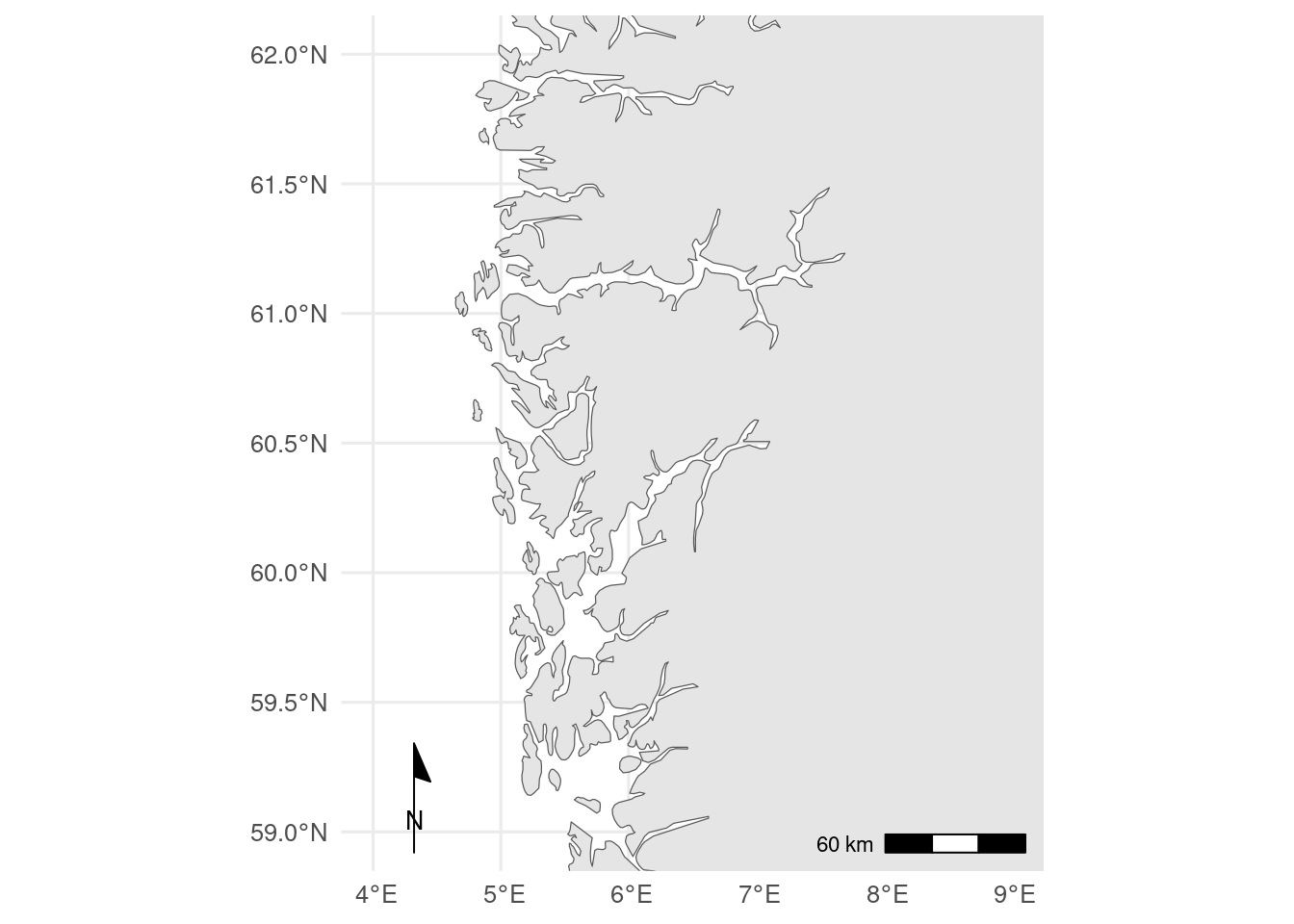## 23.5 Hints for maps

Keep it simple. Remove unnecessary features (do you really need to show the bathymetry?) and use appropriate scale data for the base map (too high resolution takes a long time to plot and can look worse).

Use facets as necessary (different species, different years etc).

If you need multiple colour scales, the `ggnewscale` package can help. Use inset maps (Chapter 22) to show your location in context.

## 23.6 Projections

The Earth is an oblate sphere and needs projecting to plot in two dimensions. This inevitably leads to distortions, especially for maps with a large extent. Different projections have different properties and may be suitable for different purposes or regions. `ggOceanMaps` can automatically select a projection based on the location, otherwise the map projection can be set using `coord_sf()`.

The projection wizard can help choose a projection for a given area (copy the PROJ string).

``````world <- ne_countries(scale = "medium", returnclass = "sf")

default <- ggplot(world) + geom_sf()

mollweide <- ggplot(world) + # Equal-area world map projection
geom_sf() +
coord_sf(crs = "+proj=moll") # projection specified with a 'proj' string

sf_use_s2(FALSE) # might need to turn spherical geometry off for some projections``````
``Spherical geometry (s2) switched off``
``````polar_lambert <-  world |>
# !important - don't crop to tightly or crop lines will show in plot
st_crop(y = c(xmin = -180, ymin = 50, xmax = 180, ymax = 90)) |>
ggplot() + # Transverse cylindrical equal-area
geom_sf() +
# projection specified with a proj string
coord_sf(crs = '+proj=laea +lon_0=14.4140625 +lat_0=90 +datum=WGS84 +units=m +no_defs',
ylim = c(100000, -3500000), # xlim/ylim in units of projection
xlim = c(-2000000, 2000000)) # here in metres``````
``````although coordinates are longitude/latitude, st_intersection assumes that they
are planar``````
``````Warning: attribute variables are assumed to be spatially constant throughout
all geometries``````
``default/mollweide/polar_lambert``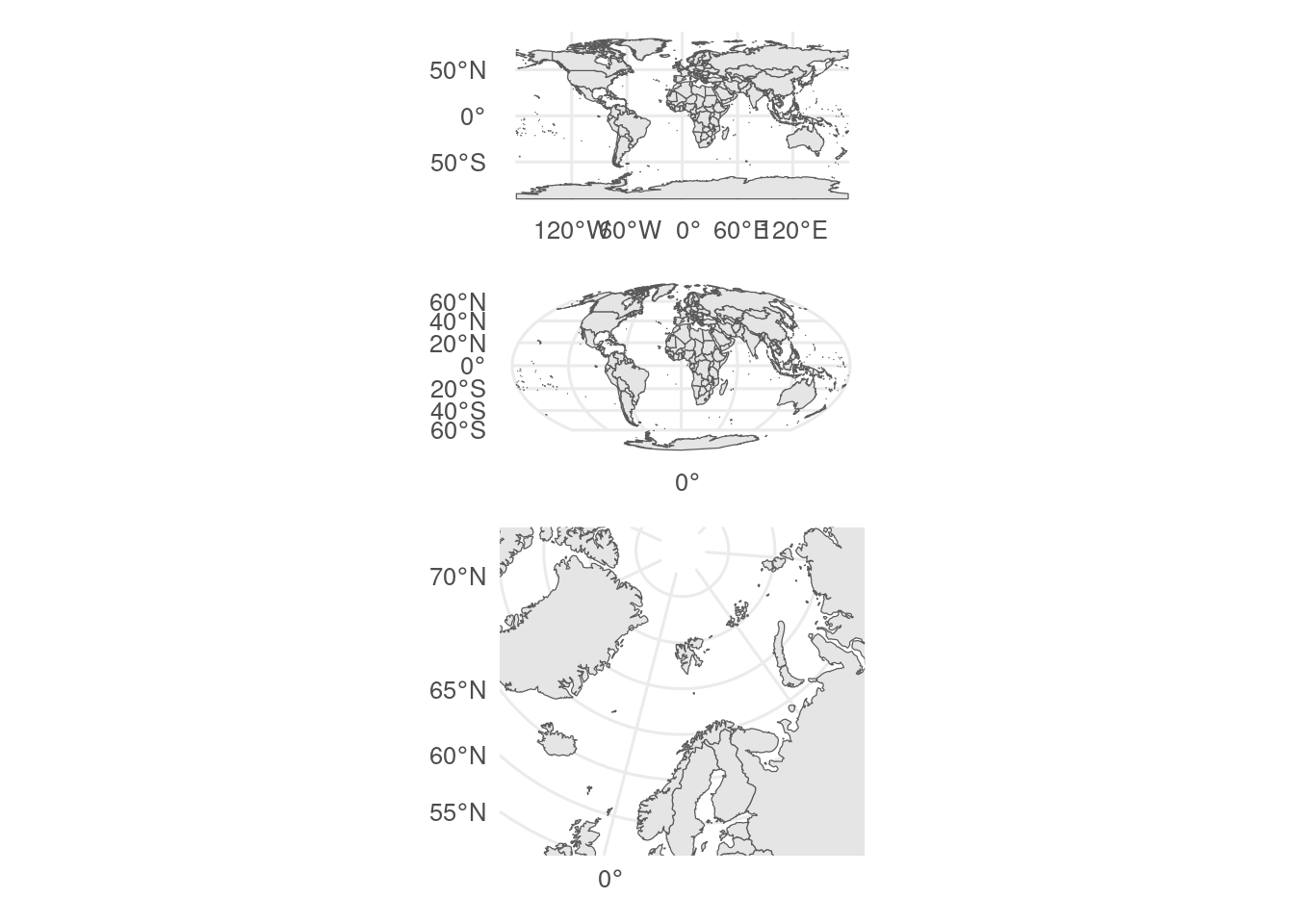Exercise

Change the projection of one of the small-scale maps you made previously.

## 23.7 Interactive maps

Interactive maps are traditionally used in theses and papers, but can be very useful on webpages and shiny apps.

The `leaflet` package is good for fully interactive maps.

``````library(leaflet) # NB uses pipes |> not +

leaflet() |>  # initialise map
setView(lng = 5.3, lat = 60.4, zoom = 9) |> # set initial map area